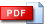# MATH104 Mathematics for Business & Economics II

### The Aim of the Course

Matrices and determinants; Applications. Solution of systems of linear equations; Inverse matrix method, Cramer's rule. Rate of change. Derivatives. Higher order derivatives. Curve sketching. Optimization. Revenue, cost, profit applications. Cost-benefit analysis. Functions of several variables. Partial derivatives. Applications. Lagrange multipliers. Integrals. Definite Integrals. Areas, Applications.

### Instructors

Asst. Prof. N. Şemi (01, 02), Dr. F. B. Rizaner (03), Dr. H. Kaffaoğlu (04)

### Tutorials

 Tutorial 1 - FunctionsTutorial 2 - Limits, Continuity and the Derivative of FunctionsTutorial 3 - Chain Rule, Tangent Line, First derivative and Second derivative TestsTutorial 4 - Applications of DerivativesTutorial 5 - Application of the Derivatives and ElasticityTutorial 6 - Bivariate functions, Cross Elasticity and LagrangeTutorial 7 - Indefinite and Definite integrals, Producer's and Consumer's Surplus### Sample Exam & Quiz Questions and Solutions

 Midterm2 Exam, Fall 2017-18Midterm2 Exami Spring  2017-18Midterm1 Exam, Fall 2018-19Midterm1 Exam, Spring 2006-07Final Examination, Spring 2006-07Quiz I, Spring 2007-08Quiz II, Spring 2007-08Quiz I, Spring 2006-07Quiz II, Spring 2006-07Quiz III, Spring 2006-07Assignment Solutions